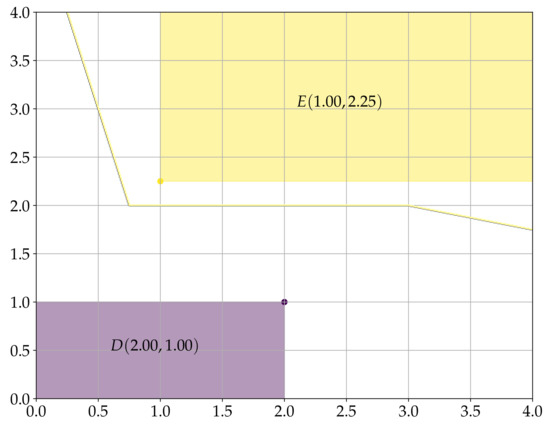Next Article in Journal
Gene-Similarity Normalization in a Genetic Algorithm for the Maximum k-Coverage Problem
Previous Article in Journal
On a Periodic Capital Injection and Barrier Dividend Strategy in the Compound Poisson Risk Model
Previous Article in Special Issue
WINkNN: Windowed Intervals’ Number kNN Classifier for Efficient Time-Series Applications
Open AccessArticle

# Reduced Dilation-Erosion Perceptron for Binary Classification

Department of Applied Mathematics, University of Campinas, Campinas 13083-859, Brazil
Mathematics 2020, 8(4), 512; https://doi.org/10.3390/math8040512
Received: 3 March 2020 / Revised: 27 March 2020 / Accepted: 30 March 2020 / Published: 2 April 2020
Dilation and erosion are two elementary operations from mathematical morphology, a non-linear lattice computing methodology widely used for image processing and analysis. The dilation-erosion perceptron (DEP) is a morphological neural network obtained by a convex combination of a dilation and an erosion followed by the application of a hard-limiter function for binary classification tasks. A DEP classifier can be trained using a convex-concave procedure along with the minimization of the hinge loss function. As a lattice computing model, the DEP classifier assumes the feature and class spaces are partially ordered sets. In many practical situations, however, there is no natural ordering for the feature patterns. Using concepts from multi-valued mathematical morphology, this paper introduces the reduced dilation-erosion (r-DEP) classifier. An r-DEP classifier is obtained by endowing the feature space with an appropriate reduced ordering. Such reduced ordering can be determined using two approaches: one based on an ensemble of support vector classifiers (SVCs) with different kernels and the other based on a bagging of similar SVCs trained using different samples of the training set. Using several binary classification datasets from the OpenML repository, the ensemble and bagging r-DEP classifiers yielded mean higher balanced accuracy scores than the linear, polynomial, and radial basis function (RBF) SVCs as well as their ensemble and a bagging of RBF SVCs. View Full-Text
Show FiguresFigure 1

MDPI and ACS Style

Valle, M.E. Reduced Dilation-Erosion Perceptron for Binary Classification. Mathematics 2020, 8, 512.